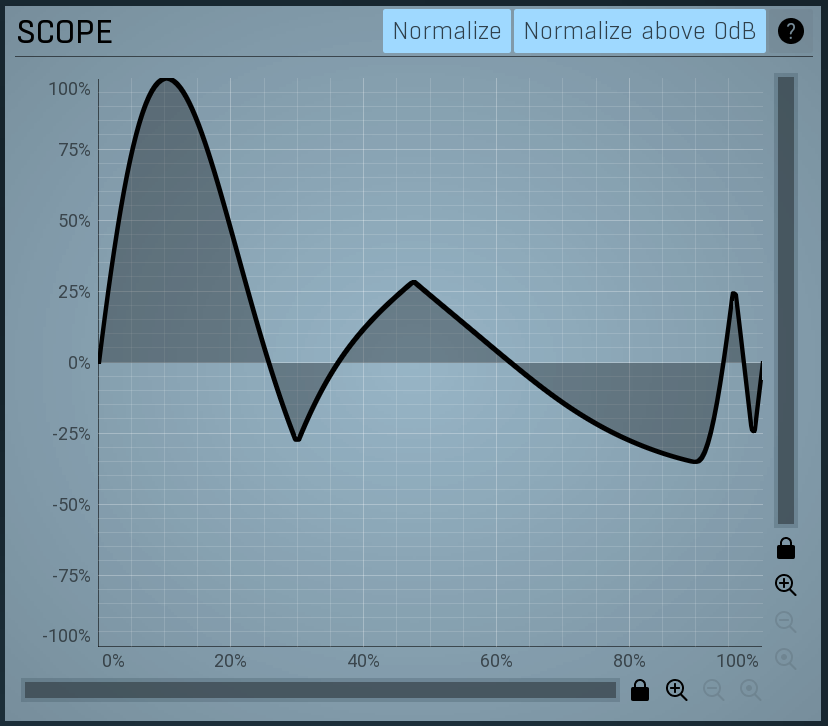Posted on

# For Sample Creators: How to use the Wavefolder and Waveshaper effectsDecent Sampler v1.7.3 introduces the new wave folder and wave shaper effects. These can be used to add extra harmonic content to your signals (aka distortion). What both of these effects have in common is that they usually sound much better when applied to a single voice rather than to an entire signal. In Decent Sampler, it is possible to apply effects at the voice level by attaching them to groups. Since each group is triggered independently, they do not share effects. In other words, each time you hit a key, a new copy of that voice will be created.

## Wave folder

The wave_folder effect allows you to fold a waveform back on itself. This is very useful for generating additional harmonic content. Here is what that looks like in practice:

These are the parameters that can be controlled:

Because wave folding tends to sound better when applied on a per-voice basis, it usually makes sense to set up the wave folder at the group level (separate group effects get created for each keypress). Example:

```.wp-block-code {
border: 0;
}

.wp-block-code > span {
display: block;
overflow: auto;
}

.shcb-language {
border: 0;
clip: rect(1px, 1px, 1px, 1px);
-webkit-clip-path: inset(50%);
clip-path: inset(50%);
height: 1px;
margin: -1px;
overflow: hidden;
position: absolute;
width: 1px;
word-wrap: normal;
word-break: normal;
}

.hljs {
box-sizing: border-box;
}

.hljs.shcb-code-table {
display: table;
width: 100%;
}

.hljs.shcb-code-table > .shcb-loc {
color: inherit;
display: table-row;
width: 100%;
}

.hljs.shcb-code-table .shcb-loc > span {
display: table-cell;
}

.wp-block-code code.hljs:not(.shcb-wrap-lines) {
white-space: pre;
}

.wp-block-code code.hljs.shcb-wrap-lines {
white-space: pre-wrap;
}

.hljs.shcb-line-numbers {
border-spacing: 0;
counter-reset: line;
}

.hljs.shcb-line-numbers > .shcb-loc {
counter-increment: line;
}

.hljs.shcb-line-numbers .shcb-loc > span {
}

.hljs.shcb-line-numbers .shcb-loc::before {
border-right: 1px solid #ddd;
content: counter(line);
display: table-cell;
text-align: right;
-webkit-user-select: none;
-moz-user-select: none;
-ms-user-select: none;
user-select: none;
white-space: nowrap;
width: 1%;
}
```<?xml version="1.0" encoding="UTF-8"?>
<DecentSampler pluginVersion="1">
<ui>
<tab>
<labeled-knob x="180" y="40" label="Drive" type="float" minValue="1" maxValue="100" textColor="FF000000" value="1">
<binding type="effect" level="group" groupIndex="0" effectIndex="1" parameter="FX_DRIVE" translation="linear" />
</labeled-knob>
<labeled-knob x="280" y="40" label="Threshold" type="float" minValue="0" maxValue="1" value="1" textColor="FF000000">
<binding type="effect" level="group" groupIndex="0" effectIndex="1" parameter="FX_THRESHOLD" translation="linear" />
</labeled-knob>
</tab>
</ui>
<groups>
<group>
<!-- samples go here -->
<effects>
<effect type="lowpass_4pl" resonance="1" frequency="500" />
<effect type="wave_folder" drive="1" threshold="1" />
</effects>
</group>
</groups>

</DecentSampler>
```Code language: HTML, XML (xml)```

## Waveshaper

The wave_shaper effect allows you to apply standard tanh waveshaping to your input signal. Here are some examples what that looks like in practice:

There are a few parameters which can be controlled:

Because wave shaping tends to sound better when applied on a per-voice basis, it usually makes sense to set up the wave shaper at the group level (separate group effects get created for each keypress). Example:

``````<DecentSampler pluginVersion="1">
<ui>
<tab>
<labeled-knob x="180" y="40" label="Drive" type="float" minValue="0" maxValue="1000" textColor="FF000000" value="0.5473124980926514">
<binding type="effect" level="group" groupIndex="0" effectIndex="0" parameter="FX_DRIVE" translation="linear"/>
</labeled-knob>
<labeled-knob x="280" y="40" label="Boost" type="float" minValue="0" maxValue="1" value="0.328312486410141" textColor="FF000000">
<binding type="effect" level="group" groupIndex="0" effectIndex="0" parameter="FX_DRIVE_BOOST" translation="linear"/>
</labeled-knob>
<labeled-knob x="380" y="40" label="Output Lvl" type="float" minValue="0" maxValue="1" value="0.1" textColor="FF000000">
<binding type="effect" level="group" groupIndex="0" effectIndex="0" parameter="FX_OUTPUT_LEVEL" translation="linear"/>
</labeled-knob>
</tab>
</ui>
<groups>
<group>
<em><!-- Samples go here. --></em>
<effects>
<effect type="wave_shaper" drive="0.5473124980926514" driveBoost="0.328312486410141" outputLevel="0.1"/>
</effects>
</group>
</groups>
```Code language: HTML, XML (xml)```